• 本文主要分享自编码器、变分自编码器和条件变分自编码器的相关知识以及在实际实践中的应用技巧，原创不易转载请注明出处，原稿获取请关注公众号【AI机器学习与知识图谱】回复：自编码器，下面首先简单认识一下：...

作者：CHEONG

公众号：AI机器学习与知识图谱

研究方向：自然语言处理与知识图谱

本文主要分享自编码器、变分自编码器和条件变分自编码器的相关知识以及在实际实践中的应用技巧，原创不易转载请注明出处，原稿获取请关注公众号【AI机器学习与知识图谱】回复：自编码器，下面首先简单认识一下：自编码器、变分自编码器和条件变分自编码器之间的关系。

1．自编码器： 自编码器结构：Encoder将输入数据进行压缩，Decoder将其转化回输入的近似值，自编码器可以对输入进行重构，但是无法充当生成模型，变分自编码器可以用作生成模型；

2．变分自编码器： 变分自编码器通过Encoder将每个样本不是压缩到单个点，而是映射到标准正态分布上，变分自编码器为每个样本产生一个概率分布；3．条件变分自编码器： 自编码器和变分自编码器都仅仅在Encoder阶段有输入，而条件变分自编码器在Encoder和Decoder阶段都有输入，使得Decoder可以按需生成特定结果。如下图所示：生成模型的难度在于判断生成分布和真实分布的相似度，自编码器寻找的是单值映射关系，而变分自编码器寻找到的是两个概率分布之间的映射关系。

一、变分自编码器

首先从VAE思想的出发点，明白条件变分自编码器是一个生成模型，给定了真实样本X，生成这个真实样本X对应的后验概率分布P(Z|X)，假设后验概率分布P(Z|X)是服从正太分布的，这目前都是Encoder部分工作，然后在Decoder部分，从分布P(Z|X)中采样变量Z，然后再通过采样样本Z还原生成X，这样就完成了从X生成X，如下图所示：但在上述变分自编码器中存在以下两个问题：

问题1． 如果在重构X^过程中，模型使得最后的方差为0，那么就没有随机性了，模型就相当于简单的自编码模型了，不能体现生成模型的特点。

针对这个问题变分自编码器是通过让所有的P(Z|X)都向标准正态分布看齐，相当于给模型添加了高斯噪声，保证了模型具有一定的生成能力，实现方法是引入KL Loss，即为了让P(Z|X)都能逼近标准正态分布N(0,I)，计算一般正态分布和标准正态分布的KL散度作为额外的Loss，根据KL散度性质，通过最小化这个Loss，便最小化了P(Z|X)和标准正态分布N(0,I)距离问题2． 如果由真实数据X得到概率分布P(Z|X)，VAE是通过神经网络拟合出来的，网络类似于传统的seq2seq模型，如VAE中采用的也是LSTM，下面便可以看一下VAE网络结构：VAE模型结构如上图，和传统的seq2seq模型不同，VAE模型encoder的结果不再是一个明确的值，而是生成一个分布 ( u , σ ) (u, \sigma) ,再从这个分布中采样最后encoder的结果，因此相同的输入可能对应不同的encoder结果，对应不同的输出

变分自编码器VAE中变分的理解： 变分体现在引入了KL散度及其性质，通过KL散度来最小化后验分布P(Z|X)与标准正态分布之间的距离。变分自编码和条件变分自编码器中由于存在一个随机变量，因此反向传播算法无法通过随机变量来回传递梯度损失信号以达到更新模型参数，为了解决这个问题，将随机变量的参数进行分解，并通过随机梯度变分贝叶斯训练模型参数

二、条件变分自编码器CVAE

变分自编码器可以无监督的对数据样本进行建模从而通过采样生成结果，条件变分自编码器在此基础上引入了监督信息条件，从而在不同的给定条件下生成不同的结果，CVAE模型图如下所示：CVAE存在的问题： KL散度消失问题，在CVAE做生成模型时，其解码器通常是一个自回归模型实现，实际会导致训练过程出现一个问题即KL正则项会消失趋近0，在实践中这意味着学习到的特征将不再能够表达观测到的数据，针对KL消失问题很多论文提出了相关策略

三、关键词提取TextRank

TF-IDF算法可以用于关键词提取，但TF-IDF仅仅从词的统计信息出发，没有考虑词之间的语义信息，因此使用TextRank算法，TextRank是一种基于图排序的关键词提取算法，考虑到了相邻词的语义关系，TextRank算法和PageRank算法在思想上类似，PageRank的节点是网页用于对网页重要度排序，TextRank算法节点是单词，用于对词的重要程度排序。

TextRank将某一个词与其前面的N个词、以及后面的N个词均具有图相邻关系，类似于N-gram语法模型。具体实现是设置一个长度为N的滑动窗口，所有在这个窗口之内的词都视作词结点的相邻结点；则TextRank构建的词图为无向图。

TextRank算法提取关键词步骤：

Step1． 把给定的文本T按照完整句子进行分割Step2． 对于每个句子，进行分词和词性标注处理，并过滤掉停用词，只保留指定词性的单词，如名词、动词和形容词，其中 t i , j t_{i,j} 是保留后的候选关键词Step3． 构建候选关键词图G = (V,E)，其中V为节点集，由(2)生成的候选关键词组成，然后采用共现关系构造任两点之间的边，两个节点之间存在边仅当它们对应的词汇在长度为K的窗口中共现，K表示窗口大小，即最多共现K个单词

Step4． 根据上面公式，迭代传播各节点的权重，直至收敛

Step5． 对节点权重进行倒序排序，从而得到最重要的T个单词，作为候选关键词

Step6． 由上述5中得到最重要的T个单词，在原始文本中进行标记，若形成相邻词组，则组合成多词关键词

四、关键词增强的条件变分自编码器

1． 自编码器

2． 变分自编码器

3． 条件变分自编码器：cvae相对于vae来说，将无监督学习变成了有监督学习，有监督的方式是对输入句子的会编码得到一个分布 N ( u 1 , σ 1 ) N(u_1, \sigma_1) ，然后对于生成结果也生成一个分布 N ( u 2 , σ 2 ) N(u_2, \sigma_2) ，对这个两个分布引入一个KL LOSS，然后最小化loss这样实现了有监督学习

4． 基于关键词增强的条件变分自编码器

CVAE中对于输入的句子先会通过RNN得到Sentense Embeddding，每个句子都会得到一个Sentense Embedding，然后将每个Sentence Embedding拼接在一起

关键词增强方式： 在关键词增强过程中，关键词是从输出句子中提取出来的，关键词增强可以理解成利用关键词生成文章，然后利用关键词结合上文信息，这样提到预测下一句的准确度，效果提升明显

关键词和输入的句子如何结合： 将Sentense Embedding和Keyword Embedding拼接在一起传入网络中，关键词相当于提供了额外的强特征信息

五、评估指标

1．BLEU：Bilingual Evaluation Under Study，一种经典的自动生成效果评估指标，含惩罚因子的BLEU评估，如下公式所示：使用的BLEU值就是覆盖率，生成句子对原句子的覆盖率大小，不考虑n-gram的顺序

BLEU-1：1-gram：指句子切分成一个字只看一个字的覆盖率

BLEU-2：2-gram：将句子一次切分成两个字，相当于看词的覆盖率，同样不考虑顺序n-gram的BLEU值

2．Distinctness：用于测评自动生成文本中用词多样性情况的指标

3．随机变量x的熵： 熵是随机变量x不确定性的度量，x取值越多越不确定熵越大4．联合熵，从一维变量扩展到多维变量时，联合随机变量的熵5．条件熵，条件熵H(Y|X)表示在已知随机变量X的条件下随机变量Y的不确定性，条件熵 H(Y|X)相当于联合熵 H(X,Y)减去单独的熵 H(X)，即H(Y|X) = H(X,Y) −H(X)6．相对熵：也就是我们提到的KL散度，相对熵可以用来衡量两个概率分布之间的差异，当两个概率分布相同时，则相对熵为0。设p(x)和q(x)是离散随机变量x中取值的两种概率分布，则p对q的相对熵为

7．交叉熵：衡量非真实分布q(x)和真实分布p(x)相似度从这里可以看出，交叉熵和相对熵相差了 H ( p ) H(p) ，而当 p p 已知的时候， H ( p ) H(p) 是个常数，所以交叉熵和相对熵在这里是等价的，反映了分布 p p q q 之间的相似程度。

六、往期精彩

【知识图谱系列】Over-Smoothing 2020综述

【知识图谱系列】基于生成式的知识图谱预训练模型

【知识图谱系列】基于2D卷积的知识图谱嵌入

【知识图谱系列】基于实数或复数空间的知识图谱嵌入

【知识图谱系列】自适应深度和广度图神经网络模型

【知识图谱系列】知识图谱多跳推理之强化学习

【知识图谱系列】知识图谱的神经符号逻辑推理

【知识图谱系列】动态时序知识图谱EvolveGCN

【知识图谱系列】多关系神经网络CompGCN

【知识图谱系列】探索DeepGNN中Over-Smoothing问题

【知识图谱系列】知识图谱表示学习综述 | 近30篇优秀论文串讲

【知识图谱系列】动态知识图谱表示学习综述 | 十篇优秀论文导读

【面经系列】八位硕博大佬的字节之旅

【机器学习系列】机器学习中的两大学派

各大AI研究院共35场NLP算法岗面经奉上

干货 | Attention注意力机制超全综述

干货 | NLP中的十个预训练模型

干货|一文弄懂机器学习中偏差和方差

FastText原理和文本分类实战，看这一篇就够了

Transformer模型细节理解及Tensorflow实现

GPT,GPT2,Bert,Transformer-XL,XLNet论文阅读速递

机器学习算法篇：最大似然估计证明最小二乘法合理性

Word2vec, Fasttext, Glove, Elmo, Bert, Flair训练词向量教程+数据+源码

Word2vec, Fasttext, Glove, Elmo, Bert, Flair训练词向量教程+数据+源码

原稿获取请关注公众号后回复：自编码器，原创不易，有用就点个赞呀！

展开全文自然语言处理 自编码器 变分自编码器 CVAE
• #这里以最具代表性的去噪自编码器为例。#导入MNIST数据集importnumpy as npimportsklearn.preprocessing as prepimporttensorflow as tffrom tensorflow.examples.tutorials.mnist importinput_data#这里使用一种...

#这里以最具代表性的去噪自编码器为例。#导入MNIST数据集

importnumpy as npimportsklearn.preprocessing as prepimporttensorflow as tffrom tensorflow.examples.tutorials.mnist importinput_data#这里使用一种参数初始化方法xavier initialization，需要对此做好定义工作。#Xaiver初始化器的作用就是让权重大小正好合适。#这里实现的是标准均匀分布的Xaiver初始化器。

def xavier_init(fan_in, fan_out, constant=1):"""目的是合理初始化权重。

参数：

fan_in --行数；

fan_out -- 列数；

constant --常数权重，条件初始化范围的倍数。

return 初始化后的权重tensor."""low= -constant * np.sqrt(6.0 / (fan_in +fan_out))

high= constant * np.sqrt(6.0 / (fan_in +fan_out))returntf.random_uniform((fan_in, fan_out),

minval=low, maxval=high,

dtype=tf.float32)#定义一个去噪的自编码类

n_input : 输入变量数；

n_hidden : 隐含层节点数；

transfer_function: 隐含层激活函数，默认是softplus；

scale : 高斯噪声系数，默认是0.1；"""

def __init__(self, n_input, n_hidden, transfer_function=tf.nn.softplus, optimizer=tf.train.AdamOptimizer(),

scale=0.1):

self.n_input=n_input

self.n_hidden=n_hidden

self.transfer=transfer_function

self.scale=tf.placeholder(tf.float32)

self.training_scale=scale

network_weights=self._initialize_weights()

self.weights=network_weights#定义网络结构，为输入x创建一个维度为n_input的placeholder，然后

#建立一个能提取特征的隐含层。

self.x =tf.placeholder(tf.float32, [None, self.n_input])

self.weights['w1']),

self.weights['b1']))

#然后，定义训练操作作为优化器self.optimizer对损失self.cost进行优化。

#最后，创建Session，并初始化自编码器全部模型参数。

self.cost = 0.5 * tf.reduce_sum(tf.pow(tf.subtract(self.reconstruction, self.x), 2.0))

self.optimizer=optimizer.minimize(self.cost)

init=tf.global_variables_initializer()

self.sess=tf.Session()

self.sess.run(init)def_initialize_weights(self):

all_weights=dict()

all_weights['w1'] =tf.Variable(xavier_init(self.n_input, self.n_hidden))

all_weights['b1'] = tf.Variable(tf.zeros([self.n_hidden], dtype=tf.float32))

all_weights['w2'] = tf.Variable(tf.zeros([self.n_hidden, self.n_input], dtype=tf.float32))

all_weights['b2'] = tf.Variable(tf.zeros([self.n_input], dtype=tf.float32))returnall_weightsdefpartial_fit(self, X):

cost, opt= self.sess.run((self.cost, self.optimizer), feed_dict={self.x: X,

self.scale: self.training_scale})returncostdefcalc_total_cost(self, X):return self.sess.run(self.cost, feed_dict={self.x: X,

self.scale: self.training_scale})#定义一个transform函数，以便返回自编码器隐含层的输出结果，目的是提供一个接口来获取抽象后的特征。

deftransform(self, X):return self.sess.run(self.hidden, feed_dict={self.x: X,

self.scale: self.training_scale})def generate(self, hidden=None):if hidden isNone:

hidden= np.random.normal(size=self.weights["b1"])return self.sess.run(self.reconstruction, feed_dict={self.hidden: hidden})defreconstruct(self, X):return self.sess.run(self.reconstruction, feed_dict={self.x: X,

self.scale: self.training_scale})def getWeights(self): #获取隐含层的权重w1.

return self.sess.run(self.weights['w1'])def getBiases(self): #获取隐含层的偏执系数b1.

return self.sess.run(self.weights['b1'])#利用TensorFlow提供的读取示例数据的函数载入MNIST数据集。

defstandard_scale(X_train, X_test):

preprocessor=prep.StandardScaler().fit(X_train)

X_train=preprocessor.transform(X_train)

X_test=preprocessor.transform(X_test)returnX_train, X_testdefget_random_block_from_data(data, batch_size):

start_index= np.random.randint(0, len(data) -batch_size)return data[start_index:(start_index +batch_size)]

X_train, X_test=standard_scale(mnist.train.images, mnist.test.images)

n_samples=int(mnist.train.num_examples)

n_hidden=200,

transfer_function=tf.nn.softplus,

scale=0.01)for epoch inrange(training_epochs):

avg_cost=0.

total_batch= int(n_samples /batch_size)#Loop over all batches

for i inrange(total_batch):

batch_xs=get_random_block_from_data(X_train, batch_size)#Fit training using batch data

cost =autoencoder.partial_fit(batch_xs)#Compute average loss

avg_cost += cost / n_samples *batch_size#Display logs per epoch step

if epoch % display_step ==0:print("Epoch:", '%04d' % (epoch + 1), "cost=", "{:.9f}".format(avg_cost))#最后对训练完的模型进行性能测试。

print("Total cost:" + str(autoencoder.calc_total_cost(X_test)))

展开全文•     原始的自编码器可以理解为数据简单降维，而这个降维的处理过程比较特殊就是使用神经网络的方式来进行训练网络权重参数和偏置，然后在再建造从获得的压缩表示中推断原数据的差值来作为它训练的一个误差。...

自动编码机的学习包括在隐藏层上对输入信号进行压缩表示，然后在输出层尽可能地复现原始输入。
原始的自编码器可以理解为数据简单降维，而这个降维的处理过程比较特殊就是使用神经网络的方式来进行训练网络权重参数和偏置，然后在再建造从获得的压缩表示中推断原数据的差值来作为它训练的一个误差。简单的结构如：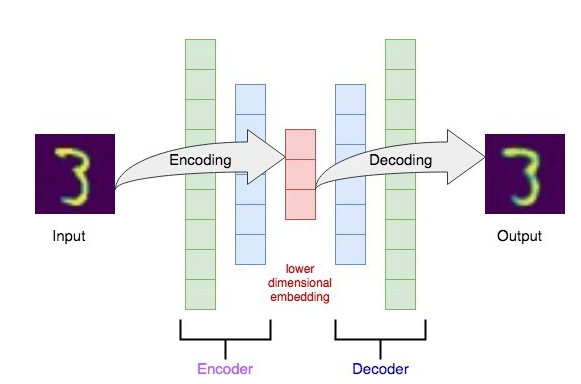注：此图来源，here.
每一层都是一个神经网络中的全连接，也就是Dense层。
而，在解码器通过学习重新推断这个原始的图片的output，这两个部分之间的差值，常常作为我们训练的优化目标，即下面这种形式：
l o s s ( X , X ^ ) = ∣ ∣ X − X ^ ∣ ∣ 2 loss(X, \hat X) = ||X - \hat X||^2
当然，是这个意思，在不同的场景中有略微的调整。
这里还是使用前面文章中我们使用过的mnist网络来进行简单的学习，如：

import tensorflow as tf
import numpy as np
import matplotlib.pyplot as plt
%matplotlib inline
import os
import struct
with open("../train-labels.idx1-ubyte", 'rb') as f:
labels = np.fromfile(f, dtype=np.uint8)

with open("../train-images.idx3-ubyte", 'rb') as f:
magic, num, rows, cols = struct.unpack('>IIII', f.read(16))
images = np.fromfile(f, dtype=np.uint8).reshape(len(labels), 784)

return images / 255., labels

image_0 = images / 255.
image_0 = np.resize(image_0, (28, 28))
#label_0 = labels
plt.imshow(image_0)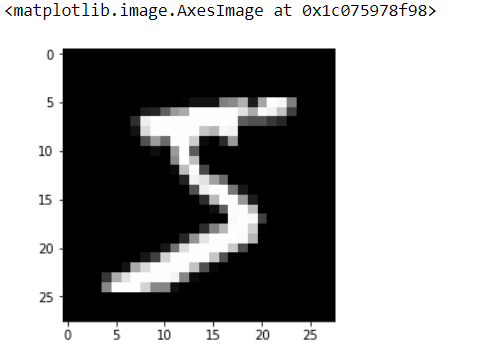然后，开始定义这个自编码器的模型，完整代码即：

# 简单的定义为只有三层，即输入层、隐藏层、输出层
input_size = len(images)
hidden_size = 64
output_size = len(images)

x = tf.keras.Input(shape=(len(images),))
y = tf.keras.layers.Dense(hidden_size, activation='relu')(x)
r = tf.keras.layers.Dense(output_size, activation='sigmoid')(y)

# 完整的自编码器
autoencoder = tf.keras.Model(inputs=x, outputs=r)

epochs = 100
batch_size = 128

history = autoencoder.fit(images, images, batch_size=batch_size, epochs=epochs, verbose=1)

# 编码
conv_encoder = tf.keras.Model(x, y)
encoded_imgs = conv_encoder.predict(images)

n = 10
plt.figure(figsize=(20, 8))
for i in range(n):
ax = plt.subplot(1, n, i+1)
plt.imshow(encoded_imgs[i].reshape(4, 16).T)
plt.gray()
ax.get_xaxis().set_visible(False)
ax.get_yaxis().set_visible(False)
plt.show()

# 解码
decoded_imgs = autoencoder.predict(images)

n = 10
plt.figure(figsize=(20, 6))
for i in range(n):
# display original
ax = plt.subplot(3, n, i+1)
plt.imshow(images[i].reshape(28, 28))
plt.gray()
ax.get_xaxis().set_visible(False)
ax.get_yaxis().set_visible(False)

# display reconstruction
ax = plt.subplot(3, n, i+n+1)
plt.imshow(decoded_imgs[i].reshape(28, 28))
plt.gray()
ax.get_xaxis().set_visible(False)
ax.get_yaxis().set_visible(False)

plt.show()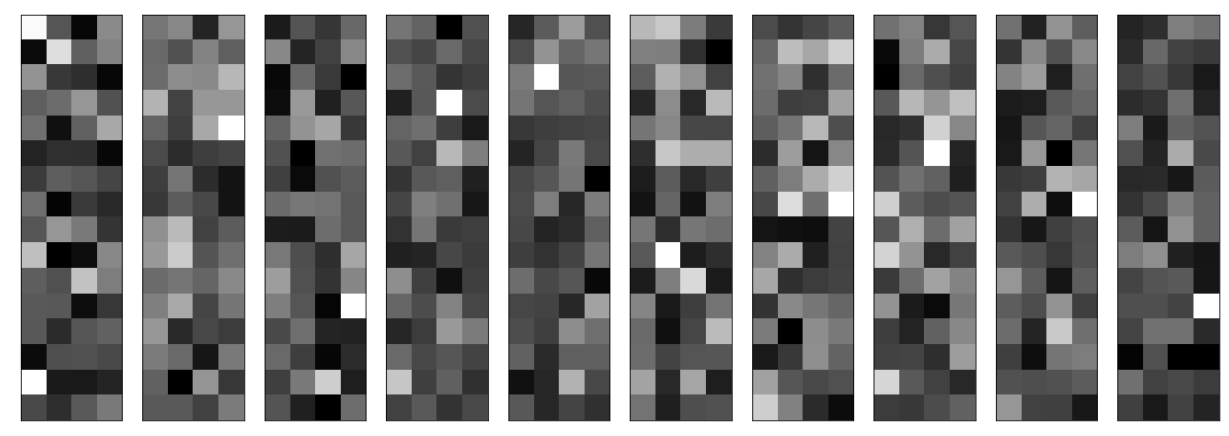上图即是编码的效果图，下图第一行是原始的图片，第二行是解码后的图片：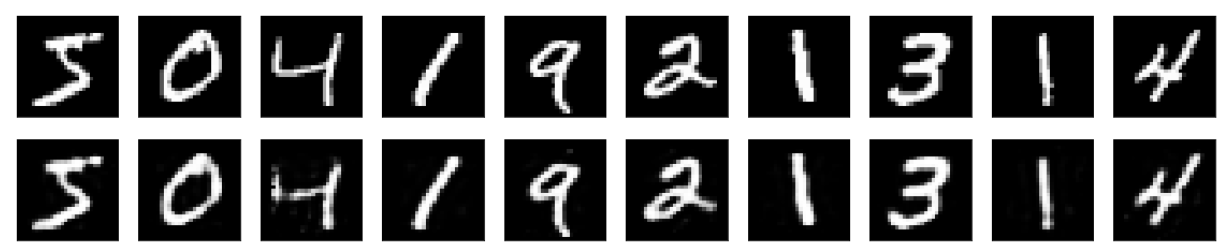展开全文自编码器 tensorflow
• 【翻译： Autoencoder Feature Extraction for Regression】 【说明：Jason BrownleePhD大神的文章个人很喜欢，所以闲暇时间里会做一点翻译和学习实践的工作，这里是相应工作的实践记录，希望能帮到有需要的人！...

【说明：Jason Brownlee PhD大神的文章个人很喜欢，所以闲暇时间里会做一点翻译和学习实践的工作，这里是相应工作的实践记录，希望能帮到有需要的人！】

自动编码器是一种神经网络，可用于学习原始数据的压缩表示。自动编码器由编码器和解码器子模型组成。编码器压缩输入，而解码器尝试根据编码器提供的压缩版本重新创建输入。训练后，将保存编码器模型，并丢弃解码器。然后，编码器可用作数据准备技术，对原始数据执行特征提取，以用于训练不同的机器学习模型。

在本教程中，您将发现如何开发和评估用于回归预测的自动编码器，完成本教程后，您将知道：

自动编码器是一种神经网络模型，可用于学习原始数据的压缩表示。
如何在训练数据集上训练自动编码器模型，并仅保存模型的编码器部分。
训练机器学习模型时如何使用编码器作为数据准备步骤。

教程概述

本教程分为三个部分： 他们是：

用于特征提取的自动编码器
回归自动编码器
自动编码器作为数据准备

用于特征提取的自动编码器

自动编码器是一种神经网络模型，旨在学习输入的压缩表示形式。它们是一种无监督的学习方法，尽管从技术上讲，它们是使用有监督的学习方法（称为自我监督）进行训练的。 通常将它们作为尝试重新创建输入的更广泛模型的一部分进行培训。

例如：X = model.predict（X）
自动编码器模型的设计通过将体系结构限制在模型中点的瓶颈处来故意使此挑战变得困难，从该瓶颈执行输入数据的重构。自动编码器的类型很多，其用途各不相同，但也许更常见的用途是作为学习型或自动特征提取模型。在这种情况下，一旦模型适合，就可以放弃模型的重建方面，可以使用直至瓶颈的模型。 模型在瓶颈处的输出是固定长度的向量，该向量提供输入数据的压缩表示。

然后，可以将来自域的输入数据提供给模型，并且可以将瓶颈处的模型输出用作监督学习模型中的特征向量，以进行可视化，或更普遍地用于降维。接下来，让我们探讨如何针对回归预测建模问题开发用于特征提取的自动编码器。

回归自动编码器

在本节中，我们将开发一种自动编码器，以学习回归预测建模问题的输入特征的压缩表示。首先，让我们定义一个回归预测建模问题。

我们将使用make_regression（）scikit-learn函数来定义具有100个输入要素（列）和1,000个示例（行）的综合回归任务。 重要的是，我们将以大多数输入变量都是冗余的（100个中的90个或90％）的方式定义问题，以便以后自动编码器可以学习有用的压缩表示形式。

下面的示例定义了数据集并总结了其形状。

# synthetic regression dataset
from sklearn.datasets import make_regression
# define dataset
X, y = make_regression(n_samples=1000, n_features=100, n_informative=10, noise=0.1, random_state=1)
# summarize the dataset
print(X.shape, y.shape)

运行示例将定义数据集并打印数组的形状，从而确认行数和列数。

(1000, 100) (1000,)

接下来，我们将开发一个多层感知器（MLP）自动编码器模型。该模型将采用所有输入列，然后输出相同的值。它将学习准确地重新创建输入模式。自动编码器由两部分组成：编码器和解码器。编码器学习如何解释输入并将其压缩为瓶颈层定义的内部表示。解码器获取编码器的输出（瓶颈层），并尝试重新创建输入。一旦对自动编码器进行了训练，解码将被丢弃，我们仅保留编码器并将其用于将输入示例压缩为瓶颈层输出的矢量。在第一个自动编码器中，我们将完全不压缩输入，而将使用与输入大小相同的瓶颈层。这应该是一个容易解决的问题，该模型将几乎完美地学习并旨在确认我们的模型已正确实现。

我们将使用功能性API定义模型。如果这是您的新手，我建议您学习本教程：

如何使用Keras功能API进行深度学习
在定义和拟合模型之前，我们将数据分为训练集和测试集，并通过将值归一化为0-1范围来缩放输入数据，这是MLP的一种良好做法。

# split into train test sets
X_train, X_test, y_train, y_test = train_test_split(X, y, test_size=0.33, random_state=1)
# scale data
t = MinMaxScaler()
t.fit(X_train)
X_train = t.transform(X_train)
X_test = t.transform(X_test)

我们将定义编码器具有一个隐藏层，该隐藏层具有与批处理归一化和ReLU激活的输入数据相同数量的节点。随后是瓶颈层，该瓶颈层的节点数与输入数据中的列数相同，例如 没有压缩。

# define encoder
visible = Input(shape=(n_inputs,))
e = Dense(n_inputs*2)(visible)
e = BatchNormalization()(e)
e = ReLU()(e)
# define bottleneck
n_bottleneck = n_inputs
bottleneck = Dense(n_bottleneck)(e)

解码器将以相同的结构定义。它将具有一层具有批处理归一化和ReLU激活的隐藏层。 输出层将具有与输入数据中的列数相同的节点数，并将使用线性激活函数输出数值。

# define decoder
d = Dense(n_inputs*2)(bottleneck)
d = BatchNormalization()(d)
d = ReLU()(d)
# output layer
output = Dense(n_inputs, activation='linear')(d)
# define autoencoder model
model = Model(inputs=visible, outputs=output)
# compile autoencoder model

考虑到重构是多输出回归问题的一种，该模型将使用高效的亚当版随机梯度下降法进行拟合，并最小化均方误差。

# compile autoencoder model

我们可以在自动编码器模型中绘制图层，以了解数据如何流过模型。

# plot the autoencoder
plot_model(model, 'autoencoder.png', show_shapes=True)

下图显示了自动编码器的图。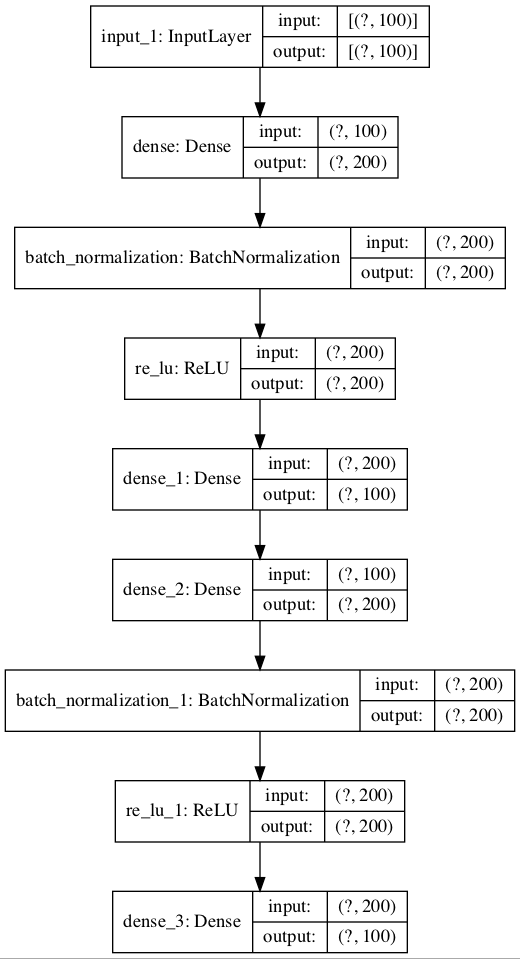接下来，我们可以训练模型以重现输入，并在保持测试集上跟踪模型的性能。 该模型经过了400个时期的训练，批次大小为16个示例。

# fit the autoencoder model to reconstruct input
history = model.fit(X_train, X_train, epochs=400, batch_size=16, verbose=2, validation_data=(X_test,X_test))

训练后，我们可以绘制火车和测试集的学习曲线，以确认模型很好地学习了重建问题。

# plot loss
pyplot.plot(history.history['loss'], label='train')
pyplot.plot(history.history['val_loss'], label='test')
pyplot.legend()
pyplot.show()

最后，如果需要，我们可以保存编码器模型供以后使用。

# define an encoder model (without the decoder)
encoder = Model(inputs=visible, outputs=bottleneck)
plot_model(encoder, 'encoder.png', show_shapes=True)
# save the encoder to file
encoder.save('encoder.h5')

作为保存编码器的一部分，我们还将绘制模型以获取瓶颈层输出形状的感觉，例如 100个元素的向量。下面提供了该图的示例。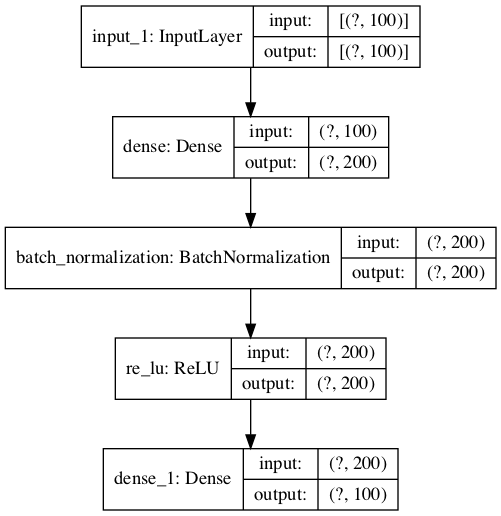综上所述，下面列出了一个自动编码器的完整示例，该示例可用于重构回归数据集的输入数据，而无需在瓶颈层进行任何压缩。

# train autoencoder for regression with no compression in the bottleneck layer
from sklearn.datasets import make_regression
from sklearn.preprocessing import MinMaxScaler
from sklearn.model_selection import train_test_split
from tensorflow.keras.models import Model
from tensorflow.keras.layers import Input
from tensorflow.keras.layers import Dense
from tensorflow.keras.layers import ReLU
from tensorflow.keras.layers import BatchNormalization
from tensorflow.keras.utils import plot_model
from matplotlib import pyplot
# define dataset
X, y = make_regression(n_samples=1000, n_features=100, n_informative=10, noise=0.1, random_state=1)
# number of input columns
n_inputs = X.shape
# split into train test sets
X_train, X_test, y_train, y_test = train_test_split(X, y, test_size=0.33, random_state=1)
# scale data
t = MinMaxScaler()
t.fit(X_train)
X_train = t.transform(X_train)
X_test = t.transform(X_test)
# define encoder
visible = Input(shape=(n_inputs,))
e = Dense(n_inputs*2)(visible)
e = BatchNormalization()(e)
e = ReLU()(e)
# define bottleneck
n_bottleneck = n_inputs
bottleneck = Dense(n_bottleneck)(e)
# define decoder
d = Dense(n_inputs*2)(bottleneck)
d = BatchNormalization()(d)
d = ReLU()(d)
# output layer
output = Dense(n_inputs, activation='linear')(d)
# define autoencoder model
model = Model(inputs=visible, outputs=output)
# compile autoencoder model
# plot the autoencoder
plot_model(model, 'autoencoder.png', show_shapes=True)
# fit the autoencoder model to reconstruct input
history = model.fit(X_train, X_train, epochs=400, batch_size=16, verbose=2, validation_data=(X_test,X_test))
# plot loss
pyplot.plot(history.history['loss'], label='train')
pyplot.plot(history.history['val_loss'], label='test')
pyplot.legend()
pyplot.show()
# define an encoder model (without the decoder)
encoder = Model(inputs=visible, outputs=bottleneck)
plot_model(encoder, 'encoder.png', show_shapes=True)
# save the encoder to file
encoder.save('encoder.h5')

运行示例符合模型，并报告沿途训练和测试集上的损失。

注意：如果在创建模型图时遇到问题，可以注释掉导入并调用plot_model（）函数。

注意：由于算法或评估程序的随机性，或者数值精度的差异，您的结果可能会有所不同。 考虑运行该示例几次并比较平均结果。

在这种情况下，我们看到损失变低了，但并没有达到零（正如我们可能预期的那样），而瓶颈层没有压缩。 也许需要进一步调整模型架构或学习超参数。

Epoch 393/400
42/42 - 0s - loss: 0.0025 - val_loss: 0.0024
Epoch 394/400
42/42 - 0s - loss: 0.0025 - val_loss: 0.0021
Epoch 395/400
42/42 - 0s - loss: 0.0023 - val_loss: 0.0021
Epoch 396/400
42/42 - 0s - loss: 0.0025 - val_loss: 0.0023
Epoch 397/400
42/42 - 0s - loss: 0.0024 - val_loss: 0.0022
Epoch 398/400
42/42 - 0s - loss: 0.0025 - val_loss: 0.0021
Epoch 399/400
42/42 - 0s - loss: 0.0026 - val_loss: 0.0022
Epoch 400/400
42/42 - 0s - loss: 0.0025 - val_loss: 0.0024

创建了一条学习曲线图，表明该模型在重构输入时取得了很好的拟合，在整个训练过程中保持稳定，而不是过度拟合。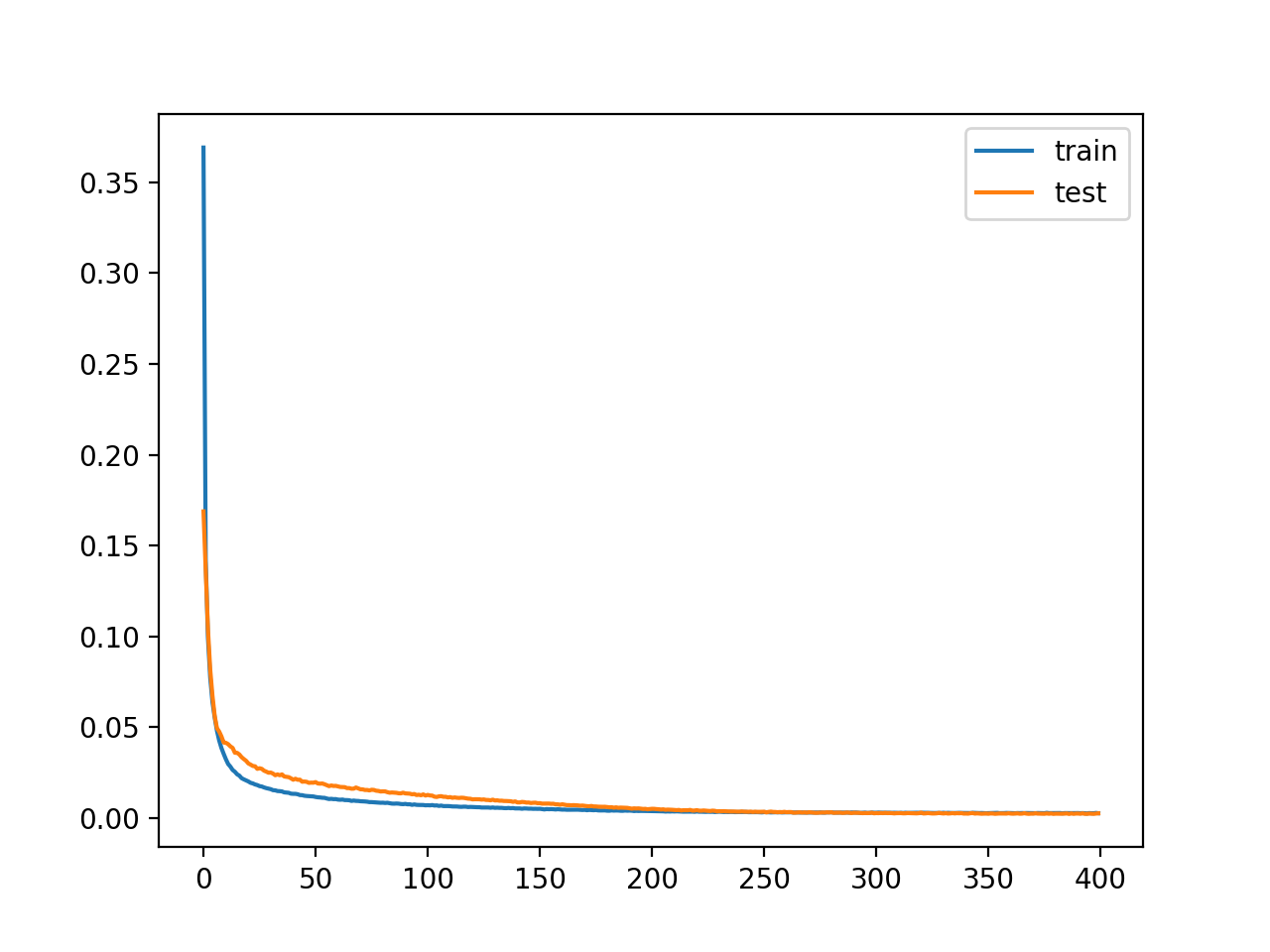到现在为止还挺好。 我们知道如何开发无压缩的自动编码器。经过训练的编码器将保存到文件“ encoder.h5”中，我们以后可以加载和使用。接下来，让我们探讨如何使用训练有素的编码器模型。

自动编码器数据准备

在本节中，我们将使用自动编码器模型中训练有素的编码器模型来压缩输入数据并训练不同的预测模型。首先，让我们为该问题建立性能基准。 这很重要，因为如果压缩编码不能提高模型的性能，那么压缩编码不会为项目增加价值，因此不应使用。我们可以直接在训练数据集上训练支持向量回归（SVR）模型，并在保留测试集上评估模型的性能。优良作法是，在拟合和评估模型之前，我们将同时缩放输入变量和目标变量。

下面列出了完整的示例。

# baseline in performance with support vector regression model
from sklearn.datasets import make_regression
from sklearn.preprocessing import MinMaxScaler
from sklearn.model_selection import train_test_split
from sklearn.svm import SVR
from sklearn.metrics import mean_absolute_error
# define dataset
X, y = make_regression(n_samples=1000, n_features=100, n_informative=10, noise=0.1, random_state=1)
# split into train test sets
X_train, X_test, y_train, y_test = train_test_split(X, y, test_size=0.33, random_state=1)
# reshape target variables so that we can transform them
y_train = y_train.reshape((len(y_train), 1))
y_test = y_test.reshape((len(y_test), 1))
# scale input data
trans_in = MinMaxScaler()
trans_in.fit(X_train)
X_train = trans_in.transform(X_train)
X_test = trans_in.transform(X_test)
# scale output data
trans_out = MinMaxScaler()
trans_out.fit(y_train)
y_train = trans_out.transform(y_train)
y_test = trans_out.transform(y_test)
# define model
model = SVR()
# fit model on the training dataset
model.fit(X_train, y_train)
# make prediction on test set
yhat = model.predict(X_test)
# invert transforms so we can calculate errors
yhat = yhat.reshape((len(yhat), 1))
yhat = trans_out.inverse_transform(yhat)
y_test = trans_out.inverse_transform(y_test)
# calculate error
score = mean_absolute_error(y_test, yhat)
print(score)

运行示例将SVR模型拟合到训练数据集上，并在测试集上对其进行评估。

注意：由于算法或评估程序的随机性，或者数值精度的差异，您的结果可能会有所不同。 考虑运行该示例几次并比较平均结果。

在这种情况下，我们可以看到该模型实现了约89的平均绝对误差（MAE）。我们希望并期望SVR模型适合输入的编码版本，以实现较低的误差，从而使编码被认为有用。

89.51082036130629

我们可以更新示例，以使用上一节中训练的编码器模型首先对数据进行编码。首先，我们可以从文件中加载经过训练的编码器模型。

# load the model from file

然后，我们可以使用编码器将原始输入数据（例如100列）转换为瓶颈向量（例如100个元素向量）。该过程可以应用于训练和测试数据集。

# encode the train data
X_train_encode = encoder.predict(X_train)
# encode the test data
X_test_encode = encoder.predict(X_test)

然后，我们可以像以前一样使用此编码数据来训练和评估SVR模型。

# define model
model = SVR()
# fit model on the training dataset
model.fit(X_train_encode, y_train)
# make prediction on test set
yhat = model.predict(X_test_encode)

完整实例如下：

# support vector regression performance with encoded input
from sklearn.datasets import make_regression
from sklearn.preprocessing import MinMaxScaler
from sklearn.model_selection import train_test_split
from sklearn.svm import SVR
from sklearn.metrics import mean_absolute_error
# define dataset
X, y = make_regression(n_samples=1000, n_features=100, n_informative=10, noise=0.1, random_state=1)
# split into train test sets
X_train, X_test, y_train, y_test = train_test_split(X, y, test_size=0.33, random_state=1)
# reshape target variables so that we can transform them
y_train = y_train.reshape((len(y_train), 1))
y_test = y_test.reshape((len(y_test), 1))
# scale input data
trans_in = MinMaxScaler()
trans_in.fit(X_train)
X_train = trans_in.transform(X_train)
X_test = trans_in.transform(X_test)
# scale output data
trans_out = MinMaxScaler()
trans_out.fit(y_train)
y_train = trans_out.transform(y_train)
y_test = trans_out.transform(y_test)
# load the model from file
# encode the train data
X_train_encode = encoder.predict(X_train)
# encode the test data
X_test_encode = encoder.predict(X_test)
# define model
model = SVR()
# fit model on the training dataset
model.fit(X_train_encode, y_train)
# make prediction on test set
yhat = model.predict(X_test_encode)
# invert transforms so we can calculate errors
yhat = yhat.reshape((len(yhat), 1))
yhat = trans_out.inverse_transform(yhat)
y_test = trans_out.inverse_transform(y_test)
# calculate error
score = mean_absolute_error(y_test, yhat)
print(score)

运行示例首先使用编码器对数据集进行编码，然后将SVR模型拟合到训练数据集上并在测试集上进行评估。

注意：由于算法或评估程序的随机性，或者数值精度的差异，您的结果可能会有所不同。 考虑运行该示例几次并比较平均结果。

在这种情况下，我们可以看到该模型实现了大约69的MAE。

这比在原始数据集上评估的同一模型更好的MAE，这表明编码对于我们选择的模型和测试工具很有帮助。

展开全文• 【翻译： Autoencoder Feature Extraction for Classification】 【说明：Jason BrownleePhD大神的文章个人很喜欢，所以闲暇时间里会做一点翻译和学习实践的工作，这里是相应工作的实践记录，希望能帮到有需要的...
• 基于自编码器的生成网络 深度自编码器( DAE) 网络，图2 给出了一个5层的深度自编码器网络示意图，其隐层( L2 ～ L4)的节点数目由多到少，再由少到多，最后输出层节点与输入层节点的数目一致。本质上， 中间的每层...神经网络 深度学习 机器学习
• 自编码器： 自编码器是用于无监督学习，高效编码的神经网络，自动编码器的目的就在于，学习一组数据的编码，通常用于数据的降维，自编码是一种无监督的算法，网络分为：输入层，隐藏层（编码层），解码层，该网络的...神经网络 深度学习 自编码器
• 自编码器的介绍1）传统自编码器2）降噪自编码器3）卷积自编码器2.自编码器的应用1）文字检索（Text Retrieval）2）相识图像检索（Similar Image Search）3）模型的预训练（Pre-training）3.自编码器的实现参考代码...自编码器 pytorch
• 自编码器(AutoEncoder)和变分自编码器(VAE) 在过去的几年中，由于一些惊人的进步，基于深度学习的生成模型越来越受到关注。依靠大量数据，精心设计的网络结构和训练技术，深度生成模型已经显示出了令人难以置信的...神经网络 机器学习 深度学习
• 此前，我介绍过一种基于自动编码器的降维方法 。然而，在那一段时间里，我专注于如何使用自动编码作为预测器，而现在我想把它们看作是一种降维技术。这篇文章的目的是解释一下自动编码器是如何工作的。它是从将原始...计算机视觉 深度学习 自编码器 自监督学习 神经网络
• 目录自编码器与变分自编码自编码器变分自编码器图变分自编码自编码器与变分自编码自编码自编码器即 Auto Encoders，简称 AE，假设存在一个神经网络： 容易发现，该神经网络的输入与输出维度相同，隐藏层...神经网络 机器学习
• 简单叙述普通自编码器、堆叠自编码器、稀疏自编码器、降噪自编码器的原理及应用。自编码器 深度学习 神经网络
• 因此，我建议：请注意，在编码器端的完全连接层之前，我有一个conv4形状层：[7, 7, 256]。在# These are the weights and biases of the mu and sigma on the encoder sidew_c_mu = tf.Variable(tf.truncated_no...
• 自动编码器（Autoencoder）是一类无监督学习的特征提取方法，它由编码器（Encoder）和解码器（Decoder）两个部分组成。其工作的原理是，首先通过编码器将初始的特征映射到一个潜在的特征空间（通常该空间的维度远...
• 自编码器结构如下图所示，编码器来实现维度的压缩，解码器实现维度的恢复重构。 自编码器可用于：训练深度学习网络、压缩、分类以及异常检测等，本文主要是异常检测的应用。 2.数据集 本文采用公开数据集，信用...python 人工智能 pytorch 深度学习 神经网络
• 编码部分负责将输入降维编码，解码部分负责让输出层通过潜在编码还原出输入层。我们的训练目标就是使得输出层与输入层之间的差距最小化。 我们会发现，有一定的风险使得训练出的AE模型是一个恒等函数，这是一.卷积 神经网络 机器学习 深度学习
• 部分内容来自：http://ufldl.stanford.edu/wiki/index.php/%E6%A0%88%E5%BC%8F%E8%87%AA%E7%BC%96%E7%A0%81%E7%AE%97%E6%B3%95栈式自编码神经网络是一个由多层稀疏自编码器组成的神经网络，其前一层自编码器的输出...栈式降噪自编码器
• 自动编码机 自动编码器就是一种尽可能复现输入信号的神经网络 为了实现这种复现，自动编码器就必须捕捉可以代表输入数据的最重要因素，和主成分分析类似，找到可以代表原信息的主要成分 一般的神经网络学习都是...机器学习 神经网络 深度学习算法 人工智能
• 自编码器是一种能够通过无监督学习，学到输入数据高效表示的神经网络。输入数据的这一高效表示（特征）称为编码（Codings），其维度一般远小于输入数据，使得自编码器可用于降维。 更重要的是，自编码器可作为强大的...
• 目录 介绍 稀疏自编码器 实例：编码器实现输入参数的重构 堆叠自编码器 ...去噪自编码器 ...实例：去噪自编码器 ...自编码器是一个前馈神经网络，尝试实现一个恒等函数，训练的时候将输出设置成与..vb6 r语言 数据分析
• 基于极限学习机的自编码器(ELM-AE) 文章目录基于极限学习机的自编码器(ELM-AE)1.算法原理2.算法实验2.1 利用ELM-AE对单维数据进行自编码2.2 利用ELM-AE对图像进行编码与还原3.参考文献4.Matlab代码 1.算法原理 ELM...算法 机器学习 python 深度学习 人工智能
• Arcgis字段如何自动编码

千次阅读 2020-12-30 16:56:55
Arcgis字段如何自动编码 1.添加字段 2.进行字段计算（可以选中部分来计算） 下面为计算方法和实现效果
• 作者｜魔王来源｜机器之心柏林工业大学深度学习方向博士生 Tilman Krokotsch 在多项任务中对比了 8 种自编码器的性能。深度学习中的自编码器。图源：https://debug...python 计算机视觉 神经网络 机器学习 人工智能
• 在这篇文章中，你将了解自编码器是如何工作的，以及为什么它们被用于医学图像去噪。 正确理解图像信息在医学等领域是至关重要的。去噪可以集中在清理旧的扫描图像上，或者有助于癌症生物学中的特征选择。噪音的存在...
• 目标函数： 第一项：自编码网络的重构误差 第二项：高斯混合模型的似然函数，可以方向更新自编码网络的参数，同时自编码网络又能更新GMM中的均值，协方差等。（为了统一朝着最小化loss的方向优化，在似然函数前添加...异常检测 无监督学习 混合高斯模型GMM 自编码器
• SPSS重新编码之自动编码功能

千次阅读 2021-03-19 10:53:07
IBM SPSS Statistics自动重新编码功能，可自动为变量创建编码，同时保留变量定义的标签和值标签，对未定义值标签的任何值，将使用原值作为重新编码后的值标签。 一、打开数据文件 首先，打开一组数据，该数据包含...
• 一、 回归语言模型 听到回归语言模型（Autoregressive LM）这个词，我们知道一般的语言模型都是从左到右计算某个词出现的概率，但是当我们做完型填空或者阅读理解这一类NLP任务的时候词的上下文信息都是需要考虑...nlp 深度学习
• 自编码器结构 既然我们已经了解了自编码器，我们就可以将上下文编码器比作自编码器。上下文编码器是一种卷积神经网络，经过训练，根据周围环境生成任意图像区域的内容：即上下文编码器接收图像区域周围的数据，并...网络 深度学习 神经网络 人工智能 机器学习...